Using the IF THEN ELSE function

Follow

The IF THEN ELSE function is a useful tool for creating a variety of calculated elements. IF THEN ELSE can be used in calculations in several different ways, including filtering, grouping, bucketing, and relabeling results. When using IF THEN ELSE, you will need to provide an element or condition to test and values if the expression passes or fails.

The IF THEN ELSE expression can be defined in two ways:

• IF (boolean condition) THEN (true value) ELSE (false value) ENDIF: The returned result will depend on whether the condition passes or fails.
• IF (boolean condition) THEN (true value) ENDIF: The returned result will always be the true result. If the conditional expression fails, results will be empty.

You can use the IF THEN ELSE function to group or bucket your results. This enables you to relabel, exclude, or segment results to indicate higher or lower values. When you combine grouping and filtering together, you can highlight results by individual values.

This section will instruct you on how to relabel your results based on the conditional expression's outcome.

You can use the IF THEN ELSE function to apply different labels to your results, depending on the outcome of your conditional expression. For example, you can use IF THEN ELSE to label whether results are above or below a metric's target. This can be useful for quickly identifying if your headline number surpassed the target goal.

This example will use the IF THEN ELSE function to indicate whether a month was above or below the target revenue. You can duplicate this example using any attribute, metric, and target.

To label results based on revenue

1. In the Calculation engine, select Standard calculated attribute.
2. Click the +Add button beneath functions.
3. Select the IF THEN ELSE function.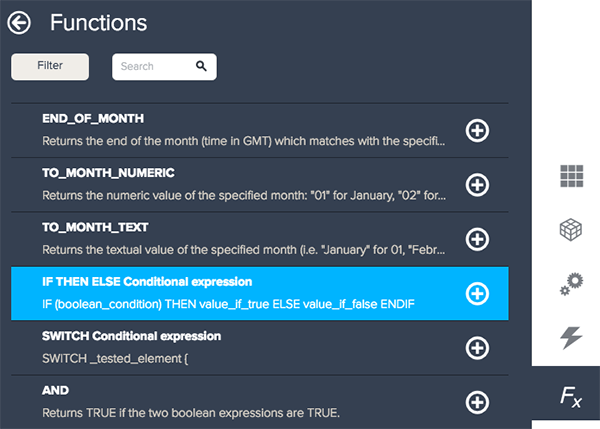4. Click inside of the _boolean_condition parentheses.
5. Select your metric from the Select a field drop-down list or type in the metric name. This example uses Revenue.
Note: If you are manually typing in a metric, you must either select BIME's auto-complete suggestion or replicate the exact format. Calculations will not process incorrect formatting.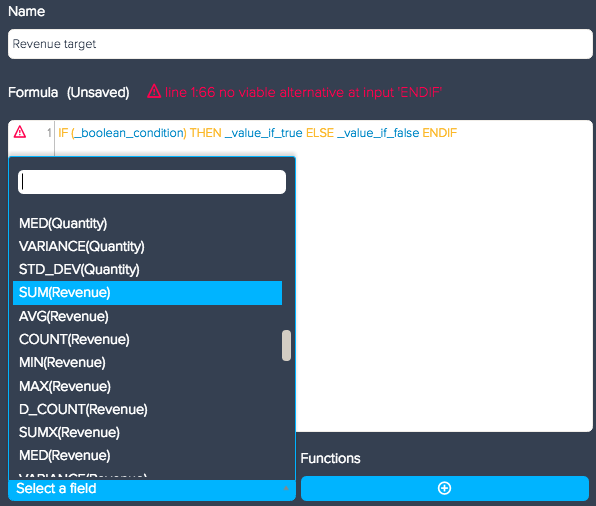6. Type in the greater than symbol (>) and your target amount.
7. For your _value_if_true enter "Over" in double quotes.
Note: You can also substitute in elements or other labels. For example, you could select your Invoice Number attribute for your _value_if_true, to view the invoice numbers for the values with revenue above 100,000.
8. For your _value_if_false enter "Under" in double quotes.9. In Computed from, select the attribute you are using in your query. If you do not select an attribute, the calculation will be measured against the total.
10. Click Save.
11. Click the + on any attribute location.
12. Select your attribute from the attributes drop-down list.

You can filter your results with IF THEN ELSE by removing the ELSE statement. When you remove the false parameter, your query will only list the true results. In most cases, it is easier to add attributes to the Filters section on the query frame, but if you want to calculate results before processing or perform unique calculations, you can filter with IF THEN ELSE.

This section incldues the following examples:

Filtering results by metric

You can filter your query to only show results that are higher or lower than a specified metric value. For example, you can limit your results to only display invoice numbers for values with revenue over 100,000. You can follow the same steps as the labeling example above, but with the following formula:

`IF (SUM(Revenue)>100000 THEN [Invoice Number] ENDIF`

When you add the attribute to your query, only the invoice numbers with revenue over 100,000 will appear.

Filtering calculations by attribute

Along with filtering results based on a metric, you can also filter calculations to a specific attribute value. This formula is useful if you want to perform calculations on one attribute value and show the original metric results or perform different calculations on the other values.

The example below calculates profit for the Accessories value in the Category attribute, but you can duplicate this example using any attribute value and calculation.

To limit calculations to an attribute value

1. In the Calculation engine menu, select Standard calculated mgetric.
2. Name your calculated metric. This example uses Accessory Profit.
3. Click the +Add button beneath Functions.
4. Select the IF THEN ELSE function.5. Click inside the _boolean_condition parentheses.
6. Select the attribute containing the restricting value from the Select a field drop-down list or type in the attribute name. This example uses Category.
Note: If you manually type in the attribute, you must either select BIME's auto-complete suggestion or replicate the exact format. Calculations will not process incorrect formatting.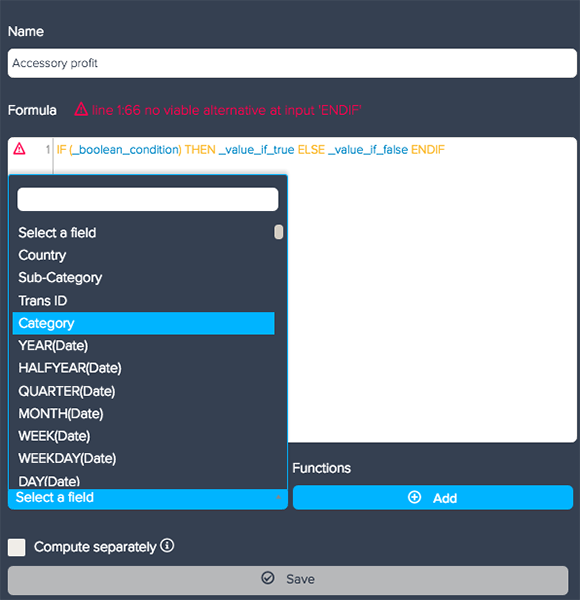7. Type in an equal sign (=) and the attribute value in "double quotes". This example uses ="Accessories".

This conditional expression will restrict your calculation to the entered attribute value.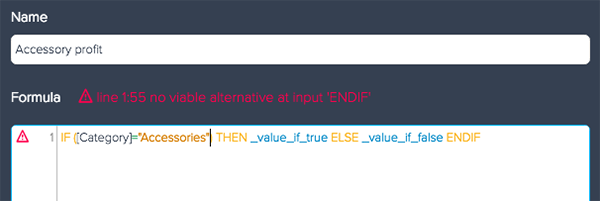8. In _value_if_true enter your calculation.
9. Delete ELSE and value_if_false. You can use the value_if_false to provide an alternative formula for results when your expression fails. This example does not want to create a false option, so the ELSE statement is deleted.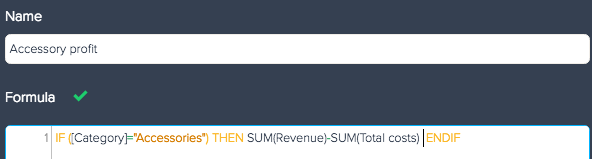10. Click Save.

Your results will be limited to only the Accessories value. If you want to create unique calculations for each attribute value, you can nest IF THEN ELSE functions to include multiple conditions (see Nesting multiple IF THEN ELSE statements .

Nesting multiple IF THEN ELSE functions

If you are using more than one ELSE IF statement in your formula, you can use ELIF to simplify your expression. For example, if you are trying to show different numbers when your revenue is greater than 100,000, equal to 100,000, or less than 100,000, you could use the ELIF expression to avoid writing multiple ELSE IF statements.

The conditional expression for this example would look like the formula below:

```IF (SUM(Revenue)>100000 THEN 1
ELIF (SUM(Revenue)=100000) THEN 2
ELIF (SUM(Revenue)<100000) THEN 3
ENDIF ```

Nesting multiple conditional IF THEN ELSE statements can be useful for creating several different groups or filtering by different conditional expressions. If you need to write several conditional expressions, you can use the SWITCH fucntion (see Using the SWITCH function).

6 out of 13 found this helpful
Have more questions? Submit a request

• The formula for Nesting multiple if then else does not seem to work.  I keep getting an error that says "Line 1:30 mismatch input '('expecting ENDIF

IF (SUM(Sales data.Total Gross Sales)>100000 THEN 1
ELIF (SUM(Sales data.Total Gross Sales)=100000) THEN 2
ELIF (SUM(Sales data.Total Gross Sales)<100000) THEN 3
ENDIF

• Hi Molly

Sorry for the delay in responding!

It looks like you are missing a ) in the first line, and might need some more ENDIFs at the end. If you retry as below, does that work? If you are making a calculated attribute you may need to 'compute from' the correct attribute to apply the condition at the condition necessary. Note that as you are using the Query Blender it will only be possible to select attributes from the primary connection.

Kind regards
Philippa

IF (SUM(Sales data.Total Gross Sales)>100000) THEN 1
ELSE IF (SUM(Sales data.Total Gross Sales)=100000) THEN 2
ELSE IF (SUM(Sales data.Total Gross Sales)<100000) THEN 3
ENDIF ENDIF ENDIF

Philippa Booth
Success Specialist
support@bime.zendesk.com